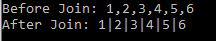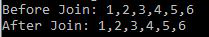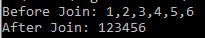# Lodash _.join() Method

• Last Updated : 03 Aug, 2020

The _.join() function is used to converts all elements in the array into a string separated by a separator.

Syntax:

```_.join(array, [separator=','])
```

Parameter: This method accepts two parameters as mentioned above and described below:

• array: It is the original array from which join operation is to be performed.
• separator: A string to separate each element of the array. If leave it by default array element separate by comma( , ).

Return Value: This function returns a string created by joining all the elements of the array using the separator.

Note: Install the lodash module by using command npm to install lodash before using the code given below.

Example 1: In this example, the function join() joins together the elements of the array into a string using ‘|’.

## Javascript

 `// Requiring the lodash library ``let lodash = require(``"lodash"``); ``   ` `// Original array to be joined ``let array = [ 1, 2, 3, 4, 5, 6 ]; ``   ` `let newArray = lodash.join(array, ``'|'``); ``console.log(``"Before Join: "` `+ array); ``   ` `// Printing newArray  ``console.log(``"After Join: "` `+ newArray);`

Output:Example 2: In this example, the function join() joins together the elements of the array into a string using ‘, ‘ since it is the default value.

## Javascript

 `// Requiring the lodash library ``let lodash = require(``"lodash"``); ``   ` `// Original array to be joined ``let array = [ 1, 2, 3, 4, 5, 6 ]; ``   ` `let newArray = lodash.join(array); ``console.log(``"Before Join: "` `+ array); ``   ` `// Printing newArray  ``console.log(``"After Join: "` `+ newArray);`

Output:Example 3: In this example, the function join() joins together the elements of the array into a string using ‘ ‘ (empty string).

## Javascript

 `// Requiring the lodash library ``let lodash = require(``"lodash"``); ``   ` `// Original array to be joined ``let array = [ 1, 2, 3, 4, 5, 6 ]; ``   ` `let newArray = lodash.join(array,``''``); ``console.log(``"Before Join: "` `+ array); ``   ` `// Printing newArray  ``console.log(``"After Join: "` `+ newArray);`

Output:My Personal Notes arrow_drop_up